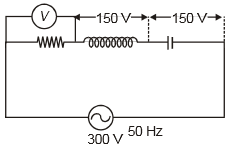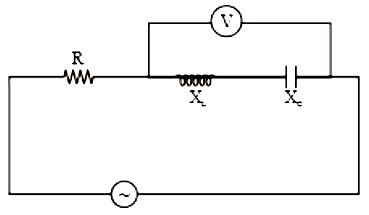In the circuit given below, the reading of the voltmeter V will be1.  0

2.  100 V

3.  200 V

4.  300 V

Concept Questions :-

Different types of AC Circuits
NEET 2021 - Achiever Batch - Aryan Raj Singh

Difficulty Level:

1.

2.

3.

4.

Concept Questions :-

Rms and average values
NEET 2021 - Achiever Batch - Aryan Raj Singh

Difficulty Level:

In a step-up transformer, the turn ratio is 1:2. A Leclanche cell (e.m.f. 1.5V) is connected across the primary coil. The voltage developed in the secondary coil would be-

1. 3.0 V

2. 0.75 V

3. 1.5 V

4. Zero

Concept Questions :-

Transformer
NEET 2021 - Achiever Batch - Aryan Raj Singh

Difficulty Level:

A 220 V, 50 Hz ac source is connected to an inductance of 0.2 H and a resistance of 20 ohms in series. What is the current in the circuit :

1.  10A

2.  5A

3.  33.3A

4.  3.33A

Concept Questions :-

Different types of AC Circuits
NEET 2021 - Achiever Batch - Aryan Raj Singh

Difficulty Level:1.

2.

3.

4.  Zero

Concept Questions :-

LC oscillations
NEET 2021 - Achiever Batch - Aryan Raj Singh

Difficulty Level:

A step-down transformer is connected to 2400 volts line and 80 amperes of current is found to flow in output load. The ratio of the turns in primary and secondary coil is 20 : 1. If transformer efficiency is 100%, then the current flowing in primary coil will be

(1) 1600 A

(2) 20 A

(3) 4 A

(4) 1.5 A

Concept Questions :-

Transformer
NEET 2021 - Achiever Batch - Aryan Raj Singh

Difficulty Level:

The potential difference V and the current i flowing through an instrument in an ac circuit of frequency f are given by $V=5\mathrm{cos}\omega \text{\hspace{0.17em}}t$ volts and I = 2 sin ωt amperes (where ω = 2πf). The power dissipated in the instrument is

(1) Zero

(2) 10 W

(3) 5 W

(4) 2.5 W

Concept Questions :-

Different types of AC Circuits
NEET 2021 - Achiever Batch - Aryan Raj Singh

Difficulty Level:

In an ac circuit, V and I are given by V = 100 sin (100 t) volts, $I=100\mathrm{sin}\text{\hspace{0.17em}}\left(100t+\frac{\pi }{3}\right)\text{\hspace{0.17em}}mA$. The power dissipated in circuit is

(1) 104 watt

(2) 10 watt

(3) 2.5 watt

(4) 5 watt

Concept Questions :-

Rms and average values
NEET 2021 - Achiever Batch - Aryan Raj Singh

Difficulty Level:

The resistance of a coil for DC is in ohms. In AC, the impedance:

(1) Will remain the same

(2) Will increase

(3) Will decrease

(4) Will be zero

Concept Questions :-

AC vs DC
NEET 2021 - Achiever Batch - Aryan Raj Singh

Difficulty Level:

A generator produces a voltage that is given by V = 240 sin 120 t, where t is in seconds. The frequency and r.m.s. voltage are

(1) 60 Hz and 240 V

(2) 19 Hz and 120 V

(3) 19 Hz and 170 V

(4) 754 Hz and 70 V

Concept Questions :-

Rms and average values